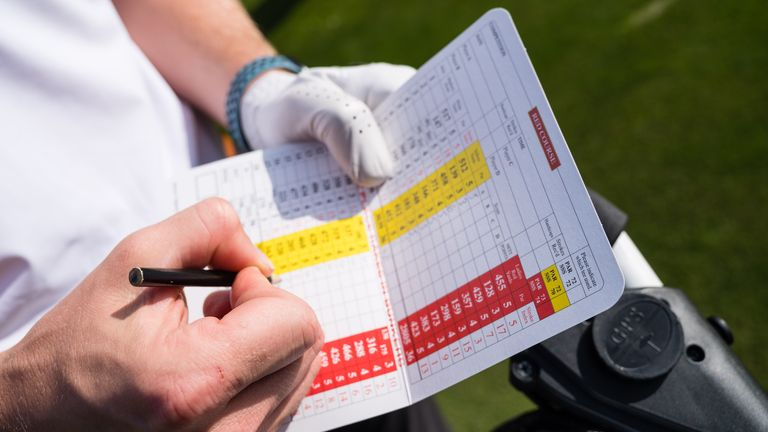# How Is Slope Rating Calculated?

Slope Rating is fundamental to the World Handicap System. Here we consider what it is and how it’s calculated.(Image credit: Tom Miles)

In this article we consider the Slope Rating. It’s a key number within the World Handicap System (WHS) used for determining a player’s Course Handicap when playing any given course off any given set of tees.

## What is Slope Rating?

Slope Rating is described by the USGA as demonstrating the “measurement of the relative playing difficulty of a course for players who are not scratch golfers, compared to scratch golfers”. The higher a course’s Slope Rating, the higher the expected difference of scoring between a scratch golfer and a bogey golfer. The Slope Rating is not purely an indication of a course’s difficulty, it is an indication of the difference in difficulty for scratch and bogey players.

## How is Slope Rating Calculated?

To determine the Slope Rating for a course requires two key numbers – Course Rating and Bogey Rating. The Course Rating is the evaluation of the playing difficulty of a golf course for the scratch player (0 handicap.) The Bogey Rating is the evaluation of the difficulty of a course for a bogey player of 20-24 handicap.

Course and Bogey Rating are calculated using the USGA Course Rating System. Course rating teams consider more than 460 variables on a standard course rating, from each set of tees. The USGA Course Rating System takes into account: the actual measured length of a golf course, factors that can affect the playing length of the course and other challenges that influence the playing difficulty of each hole (obstacle factors). Slope Rating is calculated by subtracting the Course Rating from the Bogey Rating and multiplying it by a constant.

## What Does a Slope of 113 Mean?

113 is the average Slope Rating signifying a course of standard relative difficulty. The number 113 is used in calculating a player’s Course Handicap. Each player with a WHS Handicap Index can calculate their Course Handicap for any course by dividing the Slope Rating of that course by 113 and multiplying that number by their Handicap Index.